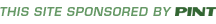NOTICE: This website is no longer updated or supported - as such many of the techniques used to build it may seem antiquated in the modern day. It is preserved for historical reasons only.

home » reference » appendix e » rgb to hexadecimal color conversion chart

# RGB to Hexadecimal Color Conversion

Setting a safe color is simply a matter of selecting a combination of safe hex values. In this case, #9966FF is a safe hex color; #9370DB is not. Most Web design tools like Macromedia Dreamweaver contain safe color pickers; so do imaging tools like Macromedia Fireworks or Adobe PhotoShop. Designers looking for color palettes, including improved color pickers and swatches, should visit http://www.visibone.com/colorlab/.

Setting an unsafe color to its nearest safe color is fairly easy—just round each particular red, green or blue value up or down to the nearest safe value. A complete conversion of hex to decimal values is shown in Table E-2. Safe values are indicated in bold.

 00=00 01=01 02=02 03=03 04=04 05=05 06=06 07=07 08=08 09=09 10=0A 11=0B 12=0C 13=0D 14=0E 15=0F 16=10 17=11 18=12 19=13 20=14 21=15 22=16 23=17 24=18 25=19 26=1A 27=1B 28=1C 29=1D 30=1E 31=1F 32=20 33=21 34=22 35=23 36=24 37=25 38=26 39=27 40=28 41=29 42=2A 43=2B 44=2C 45=2D 46=2E 47=2F 48=30 49=31 50=32 51=33 52=34 53=35 54=36 55=37 56=38 57=39 58=3A 59=3B 60=3C 61=3D 62=3E 63=3F 64=40 65=41 66=42 67=43 68=44 69=45 70=46 71=47 72=48 73=49 74=4A 75=4B 76=4C 77=4D 78=4E 79=4F 80=50 81=51 82=52 83=53 84=54 85=55 86=56 87=57 88=58 89=59 90=5A 91=5B 92=5C 93=5D 94=5E 95=5F 96=60 97=61 98=62 99=63 100=64 101=65 102=66 103=67 104=68 105=69 106=6A 107=6B 108=6C 109=6D 110=6E 111=6F 112=70 113=71 114=72 115=73 116=74 117=75 118=76 119=77 120=78 121=79 122=7A 123=7B 124=7C 125=7D 126=7E 127=7F 128=80 129=81 130=82 131=83 132=84 133=85 134=86 135=87 136=88 137=89 138=8A 139=8B 140=8C 141=8D 142=8E 143=8F 144=90 145=91 146=92 147=93 148=94 149=95 150=96 151=97 152=98 153=99 154=9A 155=9B 156=9C 157=9D 158=9E 159=9F 160=A0 161=A1 162=A2 163=A3 164=A4 165=A5 166=A6 167=A7 168=A8 169=A9 170=AA 171=AB 172=AC 173=AD 174=AE 175=AF 176=B0 177=B1 178=B2 179=B3 180=B4 181=B5 182=B6 183=B7 184=B8 185=B9 186=BA 187=BB 188=BC 189=BD 190=BE 191=BF 192=C0 193=C1 194=C2 195=C3 196=C4 197=C5 198=C6 199=C7 200=C8 201=C9 202=CA 203=CB 204=CC 205=CD 206=CE 207=CF 208=D0 209=D1 210=D2 211=D3 212=D4 213=D5 214=D6 215=D7 216=D8 217=D9 218=DA 219=DB 220=DC 221=DD 222=DE 223=DF 224=E0 225=E1 226=E2 227=E3 228=E4 229=E5 230=E6 231=E7 232=E8 233=E9 234=EA 235=EB 236=EC 237=ED 238=EE 239=EF 240=F0 241=F1 242=F2 243=F3 244=F4 245=F5 246=F6 247=F7 248=F8 249=F9 250=FA 251=FB 252=FC 253=FD 254=FE 255=FF

Although mathematically translating to the closest browser-safe color seems appropriate, it might not look correct to many people. Consider creating a hybrid color by combining multiple safe colors together. This is done simply by creating a checkerboard effect with a GIF image, in which two or more non-dithering colors are placed side by side to give the appearance of a third color. A variety of PhotoShop plug-ins such as Colorsafe (www.boxtopsoft.com) exist for mixing colors.

Next: Color Names and Numerical Equivalents(X)HTML ElementsCSS Propertiesback to top< Home | About | Chapters | Examples | Errata | Reference | Site Map > © 1997-2005 PINT Inc. [contents and programming of the site] © 2003 The McGraw-Hill Companies [contents of the print work HTML/XHTML: The Complete Reference]